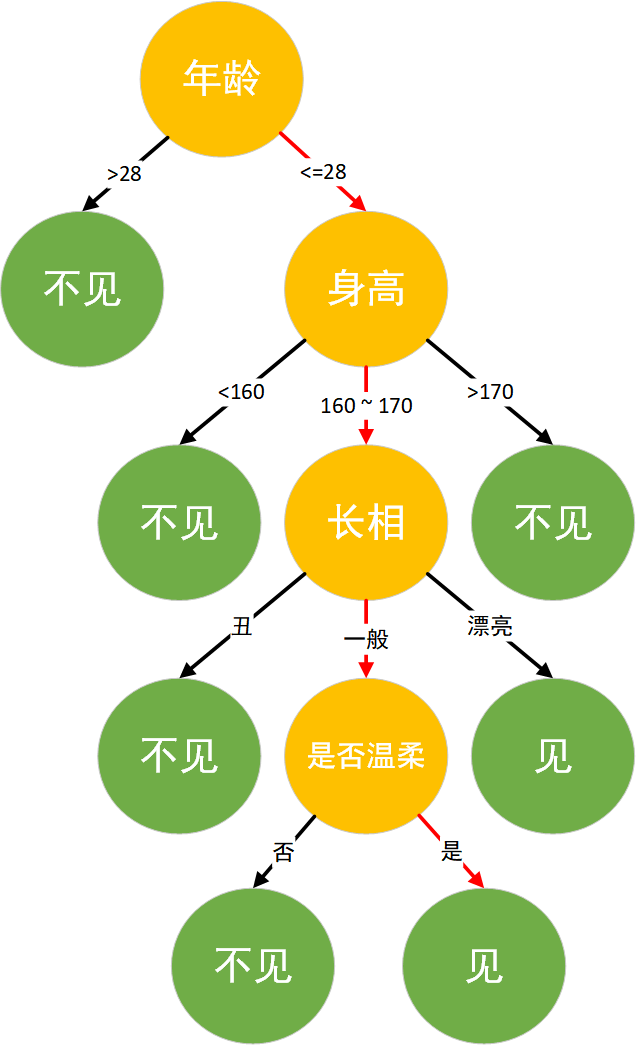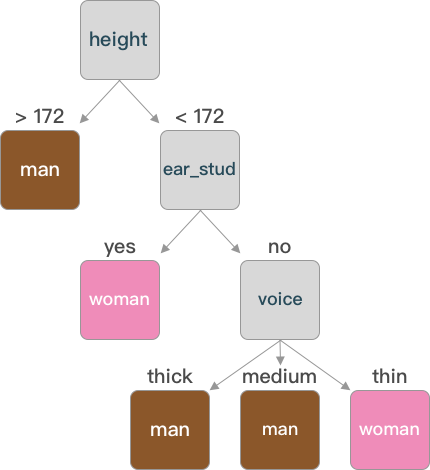## 机器学习：决策树实现与应用

6564 字 · 36 阅读 · 2023 年 05 月 11 日• 决策树算法原理
• 信息增益
• 决策树算法实现
• 学生成绩分类预测
• 决策树可视化

### 什么是决策树### 决策树算法原理

1. 数据准备 → 通过数据清洗和数据处理，将数据整理为没有缺省值的向量。
2. 寻找最佳特征 → 遍历每个特征的每一种划分方式，找到最好的划分特征。
3. 生成分支 → 划分成两个或多个节点。
4. 生成决策树 → 对分裂后的节点分别继续执行 2-3 步，直到每个节点只有一种类别。
5. 决策分类 → 根据训练决策树模型，将预测数据进行分类。

import numpy as np
import pandas as pd

def create_data():
# 生成示例数据
data_value = np.array(
[['long', 'thick', 175, 'no', 'man'],
['short', 'medium', 168, 'no', 'man'],
['short', 'thin', 178, 'yes', 'man'],
['short', 'thick', 172, 'no', 'man'],
['long', 'medium', 163, 'no', 'man'],
['short', 'thick', 180, 'no', 'man'],
['long', 'thick', 173, 'yes', 'man'],
['short', 'thin', 174, 'no', 'man'],
['long', 'thin', 164, 'yes', 'woman'],
['long', 'medium', 158, 'yes', 'woman'],
['long', 'thick', 161, 'yes', 'woman'],
['short', 'thin', 166, 'yes', 'woman'],
['long', 'thin', 158, 'no', 'woman'],
['short', 'medium', 163, 'no', 'woman'],
['long', 'thick', 161, 'yes', 'woman'],
['long', 'thin', 164, 'no', 'woman'],
['short', 'medium', 172, 'yes', 'woman']])
columns = np.array(['hair', 'voice', 'height', 'ear_stud', 'labels'])
data = pd.DataFrame(data_value.reshape(17, 5), columns=columns)
return data


data = create_data()
data


### 信息增益（ID3）

$$\operatorname{Ent}(\operatorname{D})=-\sum_{k=1}^{\left |y \right |}p_{k}\; log_{2}p_{k} \tag{1}$$

$$\operatorname{Ent}(\operatorname{data})=-\sum_{k=1}^{2}p_{k}\;log_{2}p_{k}=-(\frac{8}{17}log_{2}\frac{8}{17}+\frac{9}{17}log_{2}\frac{9}{17})=0.9975$$

import math

def get_Ent(data):
"""
参数:
data -- 数据集

返回:
Ent -- 信息熵
"""
# 计算信息熵
num_sample = len(data)  # 样本个数
label_counts = {}  # 初始化标签统计字典
for i in range(num_sample):
each_data = data.iloc[i, :]
current_label = each_data["labels"]  # 得到当前元素的标签（label）

# 如果标签不在当前字典中，添加该类标签并初始化 value=0,否则该类标签 value+1
if current_label not in label_counts.keys():
label_counts[current_label] = 0
label_counts[current_label] += 1

Ent = 0.0  # 初始化信息熵
for key in label_counts:
prob = float(label_counts[key])/num_sample
Ent -= prob * math.log(prob, 2)  # 应用信息熵公式计算信息熵
return Ent


base_ent = get_Ent(data)
base_ent


$$\operatorname{Gain}(\operatorname{D},x)=\operatorname{Ent}(\operatorname{D})- \sum_{m=1}^{M}\frac{\left | \operatorname{D}^{m}\right |}{\left | \operatorname{D} \right |}\operatorname{Ent}(\operatorname{D}^{m}) \tag{2}$$

$$\operatorname{Ent}(\operatorname{D}^{1})=-(\frac{3}{9}\;log_{2}\frac{3}{9}+\frac{6}{9}\;log_{2}\frac{6}{9})=0.449$$
$$\operatorname{Ent}(\operatorname{D}^{2})=-(\frac{5}{8}\;log_{2}\frac{5}{8}+\frac{3}{8}\;log_{2}\frac{3}{8})=0.486$$

$$\operatorname{Gain}(\operatorname{D}, \text {hair})=\operatorname{Ent}(\operatorname{D})-\sum_{m=1}^{2} \frac{\left|\operatorname{D}^{m}\right|}{|\operatorname{D}|} \operatorname{Ent}\left(\operatorname{D}^{m}\right)=0.9975-\left(\frac{9}{17} * 0.449+\frac{8}{17} * 0.486\right)=0.062$$

def get_gain(data, base_ent, feature):
"""
参数:
data -- 数据集
base_ent -- 根节点的信息熵
feature -- 计算信息增益的特征

返回:
Ent -- 信息熵
"""
# 计算信息增益
feature_list = data[feature]  # 得到一个特征的全部取值
unique_value = set(feature_list)  # 特征取值的类别
feature_ent = 0.0

for each_feature in unique_value:
temp_data = data[data[feature] == each_feature]
weight = len(temp_data)/len(feature_list)  # 计算该特征的权重值
temp_ent = weight*get_Ent(temp_data)
feature_ent = feature_ent+temp_ent

gain = base_ent - feature_ent  # 信息增益
return gain


get_gain(data,base_ent,'hair')


### 信息增益率（C4.5）

$$\operatorname{GainRatio}(\operatorname{D},a)=\frac{\operatorname{Gain}(\operatorname{D},a)}{\operatorname{IV}(a)} \tag{3}$$

$$\operatorname{IV}(a)=-\sum_{m=1}^{M}\frac{\left | \operatorname{D}^{m} \right |}{\left | \operatorname{D} \right |}\; log_{2}\frac{\left | \operatorname{D}^{m} \right |}{\left | \operatorname{D} \right |} \tag{4}$$

$\operatorname{IV}(a)$ 称为特征 $a$ 的固有值，当 $a$ 的取值数目越多，则 $\operatorname{IV}(a)$ 的值通常会比较大。例如：

$$\operatorname{IV}(\operatorname{hair})= -\frac{9}{17}\; log_{2}\frac{9}{17}-\frac{8}{17}\; log_{2}\frac{8}{17}=0.998$$
$$\operatorname{IV}(\operatorname{voice})= -\frac{7}{17}\; log_{2}\frac{7}{17} -\frac{5}{17}\; log_{2}\frac{5}{17} - \frac{5}{17}\; log_{2}\frac{5}{17} = 1.566$$

### 连续值处理

$$\operatorname{Gain}(\operatorname{D}, X)=\operatorname{Ent}(\operatorname{D})-\frac{\left|\operatorname{D}t\right|}{|\operatorname{D}|}\operatorname{Ent}\left(\operatorname{D}>t\right) \tag{5}$$

def get_splitpoint(data, base_ent, feature):
"""
参数:
data -- 数据集
base_ent -- 根节点的信息熵
feature -- 需要划分的连续特征

返回:
final_t -- 连续值最优划分点
"""
# 将连续值进行排序并转化为浮点类型
continues_value = data[feature].sort_values().astype(np.float64)
continues_value = [i for i in continues_value]  # 不保留原来的索引
t_set = []
t_ent = {}

# 得到划分点 t 的集合
for i in range(len(continues_value)-1):
temp_t = (continues_value[i]+continues_value[i+1])/2
t_set.append(temp_t)

# 计算最优划分点
for each_t in t_set:
# 将大于划分点的分为一类
temp1_data = data[data[feature].astype(np.float64) > each_t]
# 将小于划分点的分为一类
temp2_data = data[data[feature].astype(np.float64) < each_t]
weight1 = len(temp1_data)/len(data)
weight2 = len(temp2_data)/len(data)
# 计算每个划分点的信息增益
temp_ent = base_ent-weight1 * \
get_Ent(temp1_data)-weight2*get_Ent(temp2_data)
t_ent[each_t] = temp_ent
print("t_ent:", t_ent)
final_t = max(t_ent, key=t_ent.get)
return final_t


final_t = get_splitpoint(data, base_ent, 'height')
final_t


### 决策树算法实现

def choice_1(x, t):
if x > t:
return ">{}".format(t)
else:
return "<{}".format(t)

deal_data = data.copy()
# 使用lambda和map函数将 height 按照final_t划分为两个类别
deal_data["height"] = pd.Series(
map(lambda x: choice_1(int(x), final_t), deal_data["height"]))
deal_data


def choose_feature(data):
"""
参数:
data -- 数据集

返回:
best_feature -- 最优的划分特征
"""
# 选择最优划分特征
num_features = len(data.columns) - 1  # 特征数量
base_ent = get_Ent(data)
best_gain = 0.0  # 初始化信息增益
best_feature = data.columns
for i in range(num_features):  # 遍历所有特征
temp_gain = get_gain(data, base_ent, data.columns[i])    # 计算信息增益
if (temp_gain > best_gain):  # 选择最大的信息增益
best_gain = temp_gain
best_feature = data.columns[i]
return best_feature  # 返回最优特征


choose_feature(deal_data)


def create_tree(data):
"""
参数:
data -- 数据集

返回:
tree -- 以字典的形式返回决策树
"""
# 构建决策树
feature_list = data.columns[:-1].tolist()
label_list = data.iloc[:, -1]
if len(data["labels"].value_counts()) == 1:
leaf_node = data["labels"].mode().values
return leaf_node            # 第一个递归结束条件：所有的类标签完全相同
if len(feature_list) == 1:
leaf_node = data["labels"].mode().values
return leaf_node   # 第二个递归结束条件：用完了所有特征
best_feature = choose_feature(data)   # 最优划分特征
tree = {best_feature: {}}
feat_values = data[best_feature]
unique_value = set(feat_values)
for value in unique_value:
temp_data = data[data[best_feature] == value]
temp_data = temp_data.drop([best_feature], axis=1)
tree[best_feature][value] = create_tree(temp_data)
return tree


tree = create_tree(deal_data)
treedef classify(tree, test):
"""
参数:
data -- 数据集
test -- 需要测试的数据

返回:
class_label -- 分类结果
"""
# 决策分类
first_feature = list(tree.keys())  # 获取根节点
feature_dict = tree[first_feature]  # 根节点下的树
labels = test.columns.tolist()
value = test[first_feature]
for key in feature_dict.keys():
if value == key:
if type(feature_dict[key]).__name__ == 'dict':  # 判断该节点是否为叶节点
class_label = classify(feature_dict[key], test)  # 采用递归直到遍历到叶节点
else:
class_label = feature_dict[key]
return class_label


test = pd.DataFrame({"hair": ["long"], "voice": ["thin"], "height": , "ear_stud": ["yes"]})
test


test["height"] = pd.Series(map(lambda x: choice_1(int(x), final_t), test["height"]))
test


classify(tree,test)


### 学生成绩分类预测

# 导入数据集并预览


• school：学生所读学校（GP，MS）
• sex：性别（F：女，M：男）
• Pstatus：父母状态（A：同居，T：分居）
• Pedu：父母学历由低到高
• reason：选择这所学校的原因（home：家庭，course：课程设计，reputation：学校地位，other：其他）
• guardian：监护人（mother：母亲，father：父亲，other：其他）
• studytime：周末学习时长
• schoolsup：额外教育支持（yes：有，no：没有）
• famsup：家庭教育支持（yes：有，no：没有）
• paid：是否上补习班（yes：是，no：否）
• higher：是否想受更好的教育（yes：是，no：否）
• internet：是否家里联网（yes：是，no：否）
• G1：一阶段测试成绩
• G2：二阶段测试成绩
• G3：最终成绩
new_data = stu_grade.iloc[:, [0, 1, 2, 3, 4, 5, 6, 8, 9, 10, 11, 14, 15, 24, 25, 26]]


def choice_2(x):
x = int(x)
if x < 5:
elif x >= 5 and x < 10:
return "medium"
elif x >= 10 and x < 15:
return "good"
else:
return "excellent"

stu_data = new_data.copy()
stu_data["G1"] = pd.Series(map(lambda x: choice_2(x), stu_data["G1"]))
stu_data["G2"] = pd.Series(map(lambda x: choice_2(x), stu_data["G2"]))
stu_data["G3"] = pd.Series(map(lambda x: choice_2(x), stu_data["G3"]))


def choice_3(x):
x = int(x)
if x > 3:
return "high"
elif x > 1.5:
return "medium"
else:
return "low"

stu_data["Pedu"] = pd.Series(map(lambda x: choice_3(x), stu_data["Pedu"]))


def replace_feature(data):
"""
参数:
data -- 数据集

返回:
data -- 将特征值替换后的数据集
"""
# 特征值替换
for each in data.columns:  # 遍历每一个特征名称
feature_list = data[each]
unique_value = set(feature_list)
i = 0
for fea_value in unique_value:
data[each] = data[each].replace(fea_value, i)
i += 1
return data


stu_data = replace_feature(stu_data)


from sklearn.model_selection import train_test_split

X_train, X_test, y_train, y_test = train_test_split(stu_data.iloc[:, :-1], stu_data["G3"],
test_size=0.3, random_state=5)



• criterion 表示特征划分方法选择，默认为 gini (在后面会讲到)，可选择为 entropy (信息增益)。
• ramdom_state 表示随机数种子，当特征特别多时 scikit-learn 为了提高效率，随机选取部分特征来进行特征选择，即找到所有特征中较优的特征。
from sklearn.tree import DecisionTreeClassifier

dt_model = DecisionTreeClassifier(criterion='entropy', random_state=34)
dt_model.fit(X_train,y_train) # 使用训练集训练模型


### 决策树可视化

pip install graphviz  # 安装 graphviz 可视化库

from sklearn.tree import export_graphviz
import graphviz

img = export_graphviz(
dt_model, out_file=None,
feature_names=stu_data.columns[:-1].values.tolist(),  # 传入特征名称
class_names=np.array(["bad", "medium", "good", "excellent"]),  # 传入类别值
filled=True, node_ids=True,
rounded=True)

graphviz.Source(img)  # 展示决策树

y_pred = dt_model.predict(X_test) # 使用模型对测试集进行预测
y_pred

from sklearn.metrics import accuracy_score

# 计算分类准确度
accuracy_score(y_test, y_pred)


### CART 决策树

CART 决策树的构建和常见的 ID3 和 C4.5 算法的流程相似，但在特征划分选择上 CART 选择了 基尼指数 作为划分标准。数据集 $\operatorname{D}$ 的纯度可用基尼值来度量：

$$\operatorname{Gini}(\operatorname{D})=\sum_{y=1}^{|y|} \sum_{k^{\prime} \neq k} p_{k} p_{k^{\prime}} \tag{7}$$

$$\operatorname{GiniValue}(\operatorname{D},a)=\sum_{m=1}^{M}\frac{\left |\operatorname{D}^{m} \right |}{\left |\operatorname{D} \right |}\operatorname{Gini}(\operatorname{D}^{m}) \tag{8}$$

### 系列文章•